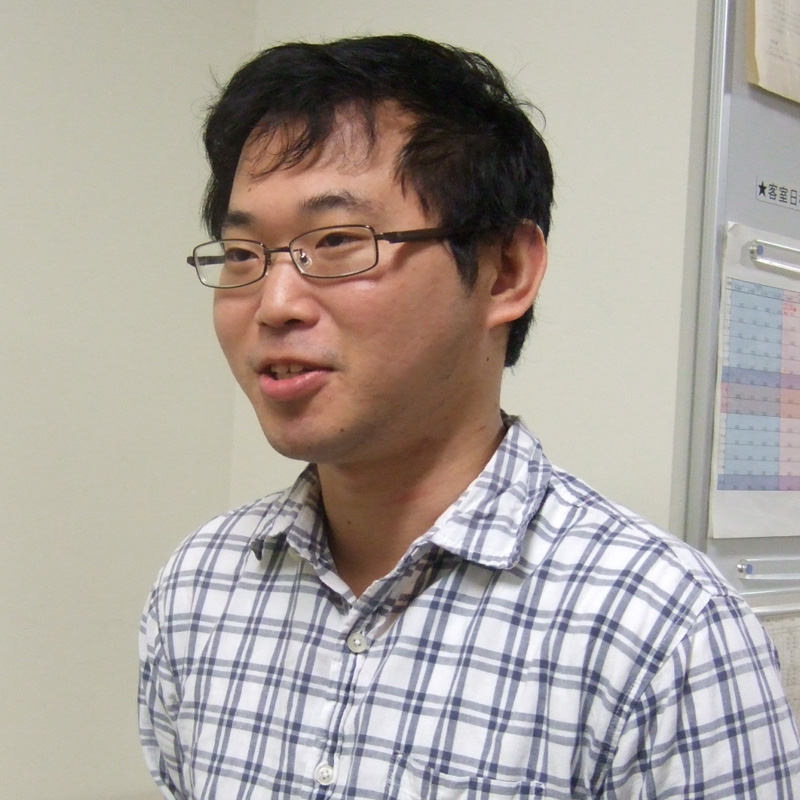## ATOBE HirakuAssociate Professor

### Approach Number Theory from the theory of Automorphic Representations

Department of Mathematics, MathematicsTheme Liftings of automorphic forms and classification of automorphic representations Field The theory of automoprhic represetations Keyword Automorhic represetations, Liftings of automorphic forms, Langlands correspondence

#### Introduction of Research

Automorphic forms, which are functions with rich symmetry, give us several number theoretic information, such as analytic continuation and functional equations of L-functions.
I study automorphic representations, which are representations consists of automorphic forms.
Arthur's classification which is a classification of automorphic representations of classical groups, is difficult to understand.
My main research theme is to study Arthur's classification using liftings of automorphic forms.

#### Representative Achievements

H. Atobe and W. T. Gan,
Local theta correspondence of tempered representations and Langlands parameters.
Invent. Math. 210 (2017), no. 2, 341–415.
H. Atobe,
On the uniqueness of generic representations in an L-packet.
Int. Math. Res. Not. IMRN 2017, no. 23, 7051–7068.
H. Atobe,
The local theta correspondence and the local Gan-Gross-Prasad conjecture for the symplectic-metaplectic case.
Math. Ann. 371 (2018), no. 1-2, 225–295.
H. Atobe, Pullbacks of Hermitian Maass lifts.
J. Number Theory 153 (2015) 158–229.
H. Atobe and W. T. Gan,
On the local Langlands correspondence and Arthur conjecture for even orthogonal groups.
Represent. Theory 21 (2017), 354–415.
 Academic degree Ph.D. Self Introduction I am from Osaka. Affiliated academic society The Mathematical Society of Japan Room address Faculty of Science, Building No. 4 4-512

Department of Mathematics, Mathematics

ATOBE Hiraku

Associate ProfessorWhat is the research theme that you are currently focusing on?

My research topic is “number theory”. In particular, I am interested in the question of whether prime numbers can be expressed in a given quadratic form. A prime number is a natural number that cannot be divided by anything other than 1 and itself, and a quadratic form is an expression such as ax2 + bxy + cy2. For example, Fermat’s theorem on sums of two squares states that an odd prime p can be expressed as p = x2 + y2 with x and y integers, if and only if p ≡ 1 mod 4. Many similar assertions have been established, but when the quadratic form is complicated, the question becomes much diﬃcult. The situation is still far from a complete solution to this problem.